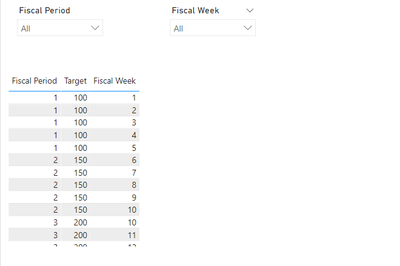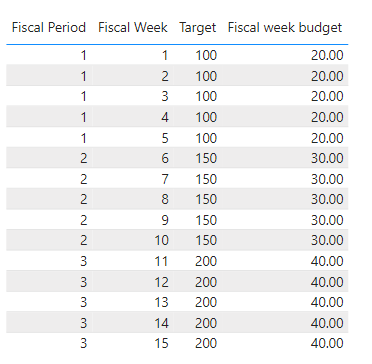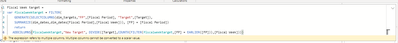cancel
Showing results for
Did you mean:Helper I

## Divide a fiscal period target by the number of fiscal weeks in the period (With Date Table)

Hi everyone.  I hav tried to crack this one but have hit a wall.

I have a report with a date table with date/ fiscal period/ fiscal weeks etc.  I also have a budget table where each period has a budget (P1,P2,P3 etc).

I want to be able to create a measure where the Period Budget is divided by the number of fiscal weeks in the period to give a Fiscal week budget.  I need this to act so I have have a visual table with Period/Fiscal Week/ Revenue/Budget.

I hae been able to create measures to work out the (Max fiscal week - Min fiscal week) +1 to work ou the number of weeks,  but when I filer te visual by period or fiscal week it breaks.

I have atached a sample file to show the tables and relationship.1 ACCEPTED SOLUTIONCommunity Support

According to your description, I create a sample.

dim_dates table:dim_target table:The two tables are related with Fiscal Period column and have a both direction.Then create a measure.

``````Fiscal week budget =
VAR _Total =
MAX ( 'dim_targets'[Target] )
VAR _MAX =
MAXX (
FILTER (
ALL ( dim_dates ),
'dim_dates'[Fiscal Period] = MAX ( 'dim_targets'[Fiscal Period] )
),
'dim_dates'[Fiscal Week]
)
VAR _MIN =
MINX (
FILTER (
ALL ( dim_dates ),
'dim_dates'[Fiscal Period] = MAX ( 'dim_targets'[Fiscal Period] )
),
'dim_dates'[Fiscal Week]
)
RETURN
DIVIDE ( _Total, _MAX - _MIN + 1 )
``````

Get the correct result.The Fiscal Period and Fiscal Week slicer can only filter the rows.I attach my sample below for your reference.

Best Regards,
Community Support Team _ kalyj

If this post helps, then please consider Accept it as the solution to help the other members find it more quickly.

4 REPLIES 4Community Support

According to your description, I create a sample.

dim_dates table:dim_target table:The two tables are related with Fiscal Period column and have a both direction.Then create a measure.

``````Fiscal week budget =
VAR _Total =
MAX ( 'dim_targets'[Target] )
VAR _MAX =
MAXX (
FILTER (
ALL ( dim_dates ),
'dim_dates'[Fiscal Period] = MAX ( 'dim_targets'[Fiscal Period] )
),
'dim_dates'[Fiscal Week]
)
VAR _MIN =
MINX (
FILTER (
ALL ( dim_dates ),
'dim_dates'[Fiscal Period] = MAX ( 'dim_targets'[Fiscal Period] )
),
'dim_dates'[Fiscal Week]
)
RETURN
DIVIDE ( _Total, _MAX - _MIN + 1 )
``````

Get the correct result.The Fiscal Period and Fiscal Week slicer can only filter the rows.I attach my sample below for your reference.

Best Regards,
Community Support Team _ kalyj

If this post helps, then please consider Accept it as the solution to help the other members find it more quickly.Helper I

Amazing @amitchandak  thank you so much.  I was actually really close with my original solution but neglected the MINX and MAXX.

Than you so much for the help and such a detailed answer!Helper I

Thank you @amitchandak  I appreciate the help.  I can't seem to get it to generate the result though.
consistantly get the error The expression refers to multiple columns. Multiple columns cannot be converted to a scalar value.

I am unsure where the extra column reference is?Super User

Var _tab=
filter(
generate(selectcolumns(Target,"FY",[FY Period], "Target", [Target]) , summarize(Date, date[FY Period],[FY Week])), [[FY Period] =[FY])
return
addcolumns(_tab , "New Target", divide([Target], countx(filter(_tab, [FY] = earlier([FY])), [FY Week])))

You also try to approch like

https://community.powerbi.com/t5/Community-Blog/How-to-divide-distribute-values-between-start-date-o...Announcements#### Exclusive opportunity for Women!

Join us for a free, hands-on Microsoft workshop led by women trainers for women where you will learn how to build a Dashboard in a Day!#### Power Platform Conference-Power BI and Fabric Sessions

Join us Oct 1 - 6 in Las Vegas for the Microsoft Power Platform Conference.Top Solution Authors
Top Kudoed Authors
Users online (1,632)